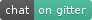# autodiff¶

autodiff is a C++17 library that uses modern and advanced programming techniques to enable automatic computation of derivatives in an efficient and easy way.

Attention

autodiff is planned to be a long-term maintained automatic differentiation project, with many more algorithms being implemented in the future. Please have in mind, however, that autodiff is still in its earlier stages of development. We welcome you to use autodiff and recommend us any improvements you think it is necessary.

## Demonstration¶

Consider the following function f(x, y, z):

```double f(double x, double y, double z)
{
return (x + y + z) * exp(x * y * z);
}
```

which we use use to evaluate variable u = f(x, y, z):

```double x = 1.0;
double y = 2.0;
double z = 3.0;
double u = f(x, y, z);
```

How can we minimally transform this code so that not only u, but also its derivatives ∂u/∂x, ∂u/∂y, and ∂u/∂z, can be computed?

The next two sections present how this can be achieved using two automatic differentiation algorithms implemented in autodiff: forward mode and reverse mode.

### Forward mode¶

In a forward mode automatic differentiation algorithm, both output variables and one or more of their derivatives are computed together. For example, the function evaluation f(x, y, z) can be transformed in a way that it will not only produce the value of u, the output variable, but also one or more of its derivatives (∂u/∂x, ∂u/∂y, ∂u/∂z) with respect to the input variables (x, y, z).

Enabling forward automatic differentiation for the calculation of derivatives using autodiff is relatively simple. For our previous function f, we only need to replace the floating-point type `double` to `autodiff::dual` for both input and output variables:

```dual f(const dual& x, const dual& y, const dual& z)
{
return (x + y + z) * exp(x * y * z);
}
```

We can now compute the derivatives ∂u/∂x, ∂u/∂y, and ∂u/∂z as follows:

```dual x = 1.0;
dual y = 2.0;
dual z = 3.0;
dual u = f(x, y, z);

double ux = derivative(f, wrt(x), at(x, y, z));
double uy = derivative(f, wrt(y), at(x, y, z));
double uz = derivative(f, wrt(z), at(x, y, z));
```

The auxiliary function `autodiff::wrt`, an acronym for with respect to, is used to indicate which input variable (x, y, z) is the selected one to compute the partial derivative of f. The auxiliary function `autodiff::at` is used to indicate where (at which values of its parameters) the derivative of f is evaluated.

### Reverse mode¶

In a reverse mode automatic differentiation algorithm, the output variable of a function is evaluated first. During this function evaluation, all mathematical operations between the input variables are "recorded" in an expression tree. By traversing this tree from top-level (output variable as the root node) to bottom-level (input variables as the leaf nodes), it is possible to compute the contribution of each branch on the derivatives of the output variable with respect to input variables.Thus, a single pass in a reverse mode calculation computes all derivatives, in contrast with forward mode, which requires one pass for each input variable. Note, however, that it is possible to change the behavior of a forward pass so that many (even all) derivatives of an output variable are computed simultaneously (e.g., in a single forward pass, ∂u/∂x, ∂u/∂y, and ∂u/∂z are evaluated together with u, in contrast with three forward passes, each one computing the individual derivatives).

Similar as before, we can use autodiff to enable reverse automatic differentiation for our function f by simply replacing type `double` by `autodiff::var` as follows:

```var f(var x, var y, var z)
{
return (x + y + z) * exp(x * y * z);
}
```

The code below demonstrates how the derivatives ∂u/∂x, ∂u/∂y, and ∂u/∂z can be calculated:

```var x = 1.0;
var y = 2.0;
var z = 3.0;
var u = f(x, y, z);

double [ux, uy, uz] = derivatives(u, wrt(x, y, z));
```

The function `autodiff::derivatives` will traverse the expression tree stored in variable `u` and compute all its derivatives with respect to the input variables (x, y, z), which are then stored in the object `dud`. The derivative of `u` with respect to input variable `x` (i.e., ∂u/∂x) can then be extracted from `dud` using `dud(x)`. The operations `dud(x)`, `dud(y)`, `dud(z)` involve no computations! Just extraction of derivatives previously computed with a call to function `autodiff::derivatives`.

## Get in touch!¶

Contact us on Gitter if you need support and assistance when using autodiff.